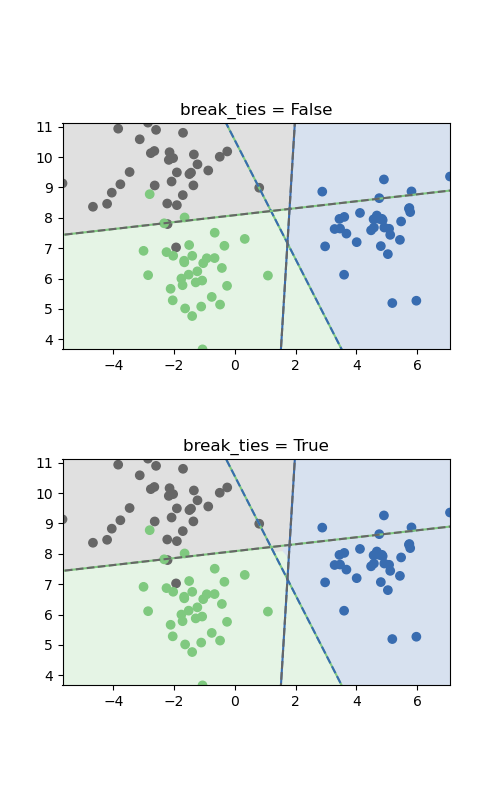# SVM Tie Breaking Example¶

Tie breaking is costly if decision_function_shape='ovr', and therefore it is not enabled by default. This example illustrates the effect of the break_ties parameter for a multiclass classification problem and decision_function_shape='ovr'.

The two plots differ only in the area in the middle where the classes are tied. If break_ties=False, all input in that area would be classified as one class, whereas if break_ties=True, the tie-breaking mechanism will create a non-convex decision boundary in that area.# Code source: Andreas Mueller, Adrin Jalali
# License: BSD 3 clause

import numpy as np
import matplotlib.pyplot as plt
from sklearn.svm import SVC
from sklearn.datasets import make_blobs

X, y = make_blobs(random_state=27)

fig, sub = plt.subplots(2, 1, figsize=(5, 8))
titles = ("break_ties = False", "break_ties = True")

for break_ties, title, ax in zip((False, True), titles, sub.flatten()):

svm = SVC(
kernel="linear", C=1, break_ties=break_ties, decision_function_shape="ovr"
).fit(X, y)

xlim = [X[:, 0].min(), X[:, 0].max()]
ylim = [X[:, 1].min(), X[:, 1].max()]

xs = np.linspace(xlim, xlim, 1000)
ys = np.linspace(ylim, ylim, 1000)
xx, yy = np.meshgrid(xs, ys)

pred = svm.predict(np.c_[xx.ravel(), yy.ravel()])

colors = [plt.cm.Accent(i) for i in [0, 4, 7]]

points = ax.scatter(X[:, 0], X[:, 1], c=y, cmap="Accent")
classes = [(0, 1), (0, 2), (1, 2)]
line = np.linspace(X[:, 1].min() - 5, X[:, 1].max() + 5)
ax.imshow(
-pred.reshape(xx.shape),
cmap="Accent",
alpha=0.2,
extent=(xlim, xlim, ylim, ylim),
)

for coef, intercept, col in zip(svm.coef_, svm.intercept_, classes):
line2 = -(line * coef + intercept) / coef
ax.plot(line2, line, "-", c=colors[col])
ax.plot(line2, line, "--", c=colors[col])
ax.set_xlim(xlim)
ax.set_ylim(ylim)
ax.set_title(title)
ax.set_aspect("equal")

plt.show()


Total running time of the script: ( 0 minutes 1.050 seconds)

Gallery generated by Sphinx-Gallery